# Integration

Integration is quite simply the opposite of differentiating. Unfortunately actually integrating isn’t that easy or much fun at all!

The first and simplest way to integrate is: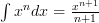$\int{x^n}{dx} = \frac{x^{n+1}}{n+1}$

That basically means add 1 to the power of the ‘$x$‘ and then divide that by the new total value of the power. Like usual the easiest way to understand this is by seeing it.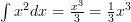$\int{x^2}{dx} = \frac{x^3}{3} = \frac{1}{3} x^3$

As with differentiation you can use any value of ‘$n$‘ and it’ll still work.

There are many different rules to integrating, and it’s best to try and remember them as they make integrating a lot easier!

Rule One

If you need to integrate a function that is mulitplied by a constant then you can move the constant outside of the integration formula, integrate the function then multiply it by the constant again.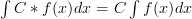$\int{C*f(x)}{dx} = C\int{f(x)}{dx}$

And here’s a quick example to help you get the gist of it: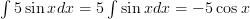$\int{5\sin x}{dx} = 5\int{\sin x}{dx} = -5\cos x$

Rule Two

If you need to integrate two functions that are being added together, then you can seperate the functions, integrate them seperately and finally add them back together.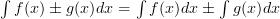$\int{f(x) \pm g(x)}{dx} = \int{f(x)}{dx} \pm \int{g(x)}{dx}$

Here’s an example for your viewing pleasure!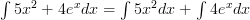$\int{5x^2 + 4e^x}{dx} = \int{5x^2}{dx} + \int{4e^x}{dx}$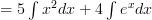$= 5\int{x^2}{dx} + 4\int{e^x}{dx}$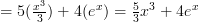$= 5(\frac{x^3}{3}) + 4(e^x) = \frac{5}{3}x^3 + 4e^x$

Rule Three

This ones a little bit harder to get your head around, but practise makes perfect! Basically you can add a constant to a function without affecting it, if ‘$x$‘ is in the first degree i.e. it has no power.

if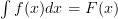$\int{f(x)}{dx} = F(x)$

then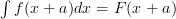$\int{f(x+a)}{dx} = F(x+a)$

Here’s how it looks with some actual numbers in: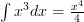$\int{x^3}{dx} = \frac{x^4}{4}$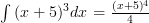$\int{(x+5)^3}{dx} = \frac{(x+5)^4}{4}$

Rule Four

This one works if ‘$a$‘ and ‘$b$‘ are constants and applied to a function of ‘$x$‘. To be honest the easiest way to understand it is by looking at this:

if$\int{f(x)}{dx} = F(x)$

then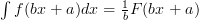$\int{f(bx+a)}{dx} = \frac{1}{b} F(bx+a)$

Still confused? I am a little bit, here’s one with numbers, see if that helps.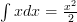$\int{x}{dx} = \frac{x^2}{2}$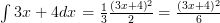$\int{3x+4}{dx} = \frac{1}{3} \frac{(3x+4)^2}{2} = \frac{(3x+4)^2}{6}$

These rules come in very useful, and sometimes you’ll need to use a mixture of these rules with the methods of integration to find the final answer.